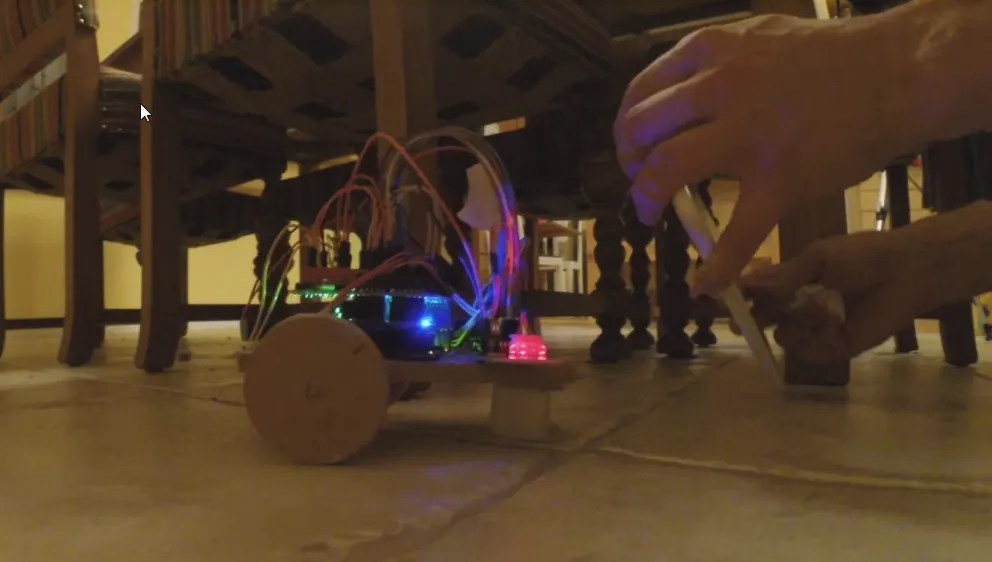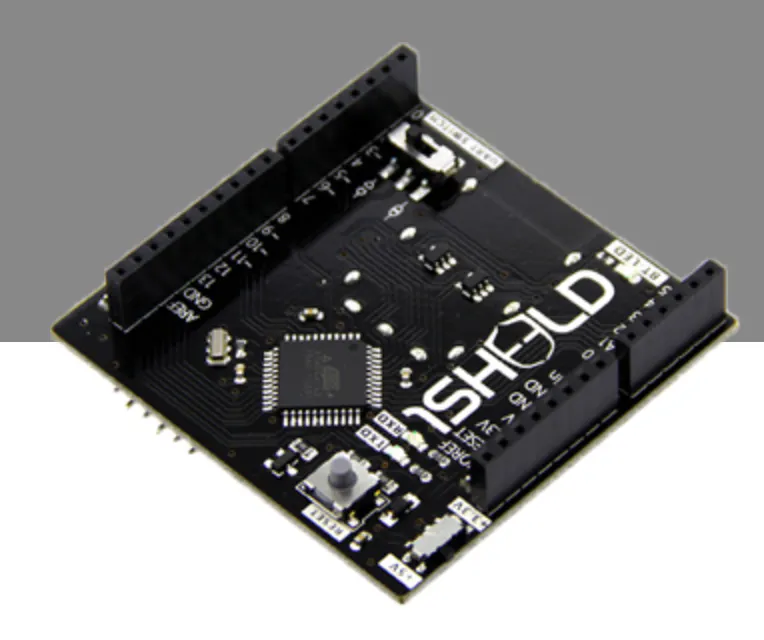Published

# Bluetooth Steering wheel for a robot using 1Sheeld

A simple bumper robot with a simple steering wheel using a 1Sheeld, and an Android tablet/phone.

BeginnerFull instructions provided2,868## Things used in this project

### Hardware components

 28BYJ-48 Stepper motors, costs around 2\$ on ebay a piece, or buy many for less.
×2
 74HC595 Shift register
×11Sheeld
×1
 Micro switch Some kind of button, these are the simplest ones, buy 100pc for about a 1\$ on ebay.com
×1Arduino UNO & Genuino UNO Can be bought a cheap China copy for around 4-6\$
×1

### Hand tools and fabrication machines

 Glue gun

## Code

### arduino_bumper_robot_595_1sheeld3.ino

C/C++
```/*
Bumper robot code written by: Kristian Blsol 6/9/2015 kristian@borgstedt.com
Original BYJ48 Stepper motor code written By :Mohannad Rawashdeh 28/9/2013, Rewritten for use with a 595 Shift Register instead by Kristian Blsol 16/9/2015.

Version 0.1 was without the 595

Version 0.2 added a 74HC595: This program is for making a really simple (and cheap) "first" robot to play with Arduino. It is a bumper robot. This particular version
Uses a 74HC595 to drive the Two motors, 28BYJ-48. This minimizes the pins used for the motors, from the original 8 to three for both motors in this
Sketch.

Version 0.2a is this code with 1Sheeld Accelerometer for turning functionality of the robot.

An
See more on
or
Duinos.net: http://duinos.net/show/?id=238 (version 0.2a)
*/

#define CUSTOM_SETTINGS
#define INCLUDE_ACCELEROMETER_SENSOR_SHIELD

#include <OneSheeld.h>

int latchPin = 2; //pin 12 on the 595
int dataPin = 3;  //pin 14 on the 595
int clockPin = 4; //pin 11 on the 595
int ledPin = 13;

#define leftBumper 8
#define rightBumper 9

int stepper1val;
int stepper2val;
int Steps1 = 0;
int Steps2 = 0;
int wheelL = 0;
int wheelR = 0;
int AndroidAngle = 0;
//defaults to forward
boolean Direction1 = false;// gre
boolean Direction2 = true;// gre
//dir1=1 dir2=0 goes backwards
//dir1=1 dir2=1 turns right
//dir1=0 dir2=0 turns left
//dir1=0 dir2=1 goes forward

unsigned long last_time;
unsigned long currentMillis ;
int steps_left1=4095; //4095 is a whole rotation
int steps_left2=4095; //4095 is a whole rotation
long time;

void setup()
{
OneSheeld.begin();
pinMode(latchPin, OUTPUT);
pinMode(dataPin, OUTPUT);
pinMode(clockPin, OUTPUT);
pinMode(ledPin, OUTPUT);
}

void loop()
{

AndroidAngle=AccelerometerSensor.getY();
//wheelL=map(AndroidAngle, -9, 0, 0, 4);
//wheelR=map(AndroidAngle, 9, 0, 0, 4);

//check bumpers
back();
turnRight();
back();
turnLeft();

//STEP1 for moving forward
currentMillis = micros();
if(currentMillis-last_time>=1000) {
//moving forward
//defaults to moving forward
Direction1 = false;
Direction2 = true;
if (AndroidAngle>-4) {stepper1(1);}
if (AndroidAngle<4) {stepper2(1);}
digitalWrite(latchPin, LOW);
shiftOut(dataPin, clockPin, MSBFIRST, stepper1val+stepper2val);
digitalWrite(latchPin, HIGH);
time=time+micros()-last_time;
last_time=micros();
}
//STEP2 for steering
currentMillis = micros();
if(currentMillis-last_time>=1000){
//moving forward
//defaults to moving forward
Direction1 = false;
Direction2 = true;
if (AndroidAngle>-6) {stepper1(1);}
if (AndroidAngle<6) {stepper2(1);}
digitalWrite(latchPin, LOW);
//if (AndroidAngle>-6) {shiftOut(dataPin, clockPin, MSBFIRST, stepper1val);}
//if (AndroidAngle<6) {shiftOut(dataPin, clockPin, MSBFIRST, stepper2val);}
shiftOut(dataPin, clockPin, MSBFIRST, stepper1val+stepper2val);
digitalWrite(latchPin, HIGH);
time=time+micros()-last_time;
last_time=micros();
}

} //void loop

void back(){
//back up a bit
steps_left1=1024;
steps_left2=1024;
while(steps_left1>0){
//dir1=1 dir2=0 goes backwards
Direction1 = true;
Direction2 = false;
currentMillis = micros();
if(currentMillis-last_time>=1000){
stepper1(1);
stepper2(1);
digitalWrite(latchPin, LOW);
shiftOut(dataPin, clockPin, MSBFIRST, stepper1val+stepper2val);
digitalWrite(latchPin, HIGH);
time=time+micros()-last_time;
last_time=micros();
steps_left1--;
steps_left2--;
}
}
}

void turnRight() {
//turn right
steps_left1=1024;
steps_left2=1024;
//dir1=1 dir2=1 turns right
while(steps_left1>0){
Direction1 = true;
Direction2 = true;
currentMillis = micros();
if(currentMillis-last_time>=1000){
stepper1(1);
stepper2(1);
digitalWrite(latchPin, LOW);
shiftOut(dataPin, clockPin, MSBFIRST, stepper1val+stepper2val);
digitalWrite(latchPin, HIGH);
time=time+micros()-last_time;
last_time=micros();
steps_left1--;
steps_left2--;
}
}
}

void turnLeft() {
//turn left
steps_left1=1024;
steps_left2=1024;
//dir1=0 dir2=0 turns left
while(steps_left1>0){
Direction1 = false;
Direction2 = false;
currentMillis = micros();
if(currentMillis-last_time>=1000){
stepper1(1);
stepper2(1);
digitalWrite(latchPin, LOW);
shiftOut(dataPin, clockPin, MSBFIRST, stepper1val+stepper2val);
digitalWrite(latchPin, HIGH);
time=time+micros()-last_time;
last_time=micros();
steps_left1--;
steps_left2--;
}
}
}

// motor 1 is 1 2 4 8
void stepper1(int xw){
for (int x=0;x<xw;x++){
switch(Steps1){
case 0:
stepper1val=8;
break;
case 1:
stepper1val=12;
break;
case 2:
stepper1val=4;
break;
case 3:
stepper1val=6;
break;
case 4:
stepper1val=2;
break;
case 5:
stepper1val=3;
break;
case 6:
stepper1val=1;
break;
case 7:
stepper1val=9;
break;
default:
stepper1val=0;
break;
}
SetDirection1();
}
}

// 16 32 64 128
void stepper2(int xw){
for (int x=0;x<xw;x++){
switch(Steps2){
case 0:
stepper2val=128;
break;
case 1:
stepper2val=192;
break;
case 2:
stepper2val=64;
break;
case 3:
stepper2val=96;
break;
case 4:
stepper2val=32;
break;
case 5:
stepper2val=48;
break;
case 6:
stepper2val=16;
break;
case 7:
stepper2val=144;
break;
default:
stepper2val=8;
break;
}
SetDirection2();
}
}

void SetDirection1(){
if(Direction1==1){ Steps1++;}
if(Direction1==0){ Steps1--; }
if(Steps1>7){Steps1=0;}
if(Steps1<0){Steps1=7; }
}
void SetDirection2(){
if(Direction2==1){ Steps2++;}
if(Direction2==0){ Steps2--; }
if(Steps2>7){Steps2=0;}
if(Steps2<0){Steps2=7; }
}
```

### Untitled file

Arduino
```/*
Bumper robot code written by: Kristian Blåsol 6/9/2015 kristian@borgstedt.com
Original BYJ48 Stepper motor code written By :Mohannad Rawashdeh 28/9/2013, Rewritten for use with a 595 Shift Register instead by Kristian Blåsol 16/9/2015.

Version 0.1 was without the 595

Version 0.2 added a 74HC595: This program is for making a really simple (and cheap) "first" robot to play with Arduino. It is a bumper robot. This particular version
Uses a 74HC595 to drive the Two motors, 28BYJ-48. This minimizes the pins used for the motors, from the original 8 to three for both motors in this
Sketch.

Version 0.2a is this code with 1Sheeld Accelerometer for turning functionality of the robot.

An
See more on
or
Duinos.net: http://duinos.net/show/?id=238 (version 0.2a)
*/

#define CUSTOM_SETTINGS
#define INCLUDE_ACCELEROMETER_SENSOR_SHIELD

#include <OneSheeld.h>

int latchPin = 2; //pin 12 on the 595
int dataPin = 3;  //pin 14 on the 595
int clockPin = 4; //pin 11 on the 595
int ledPin = 13;

#define leftBumper 8
#define rightBumper 9

int stepper1val;
int stepper2val;
int Steps1 = 0;
int Steps2 = 0;
int wheelL = 0;
int wheelR = 0;
int AndroidAngle = 0;
//defaults to forward
boolean Direction1 = false;// gre
boolean Direction2 = true;// gre
//dir1=1 dir2=0 goes backwards
//dir1=1 dir2=1 turns right
//dir1=0 dir2=0 turns left
//dir1=0 dir2=1 goes forward

unsigned long last_time;
unsigned long currentMillis ;
int steps_left1=4095; //4095 is a whole rotation
int steps_left2=4095; //4095 is a whole rotation
long time;

void setup()
{
OneSheeld.begin();
pinMode(latchPin, OUTPUT);
pinMode(dataPin, OUTPUT);
pinMode(clockPin, OUTPUT);
pinMode(ledPin, OUTPUT);
}

void loop()
{

AndroidAngle=AccelerometerSensor.getY();
//wheelL=map(AndroidAngle, -9, 0, 0, 4);
//wheelR=map(AndroidAngle, 9, 0, 0, 4);

//check bumpers
back();
turnRight();
back();
turnLeft();

//STEP1 for moving forward
currentMillis = micros();
if(currentMillis-last_time>=1000) {
//moving forward
//defaults to moving forward
Direction1 = false;
Direction2 = true;
if (AndroidAngle>-4) {stepper1(1);}
if (AndroidAngle<4) {stepper2(1);}
digitalWrite(latchPin, LOW);
shiftOut(dataPin, clockPin, MSBFIRST, stepper1val+stepper2val);
digitalWrite(latchPin, HIGH);
time=time+micros()-last_time;
last_time=micros();
}
//STEP2 for steering
currentMillis = micros();
if(currentMillis-last_time>=1000){
//moving forward
//defaults to moving forward
Direction1 = false;
Direction2 = true;
if (AndroidAngle>-6) {stepper1(1);}
if (AndroidAngle<6) {stepper2(1);}
digitalWrite(latchPin, LOW);
//if (AndroidAngle>-6) {shiftOut(dataPin, clockPin, MSBFIRST, stepper1val);}
//if (AndroidAngle<6) {shiftOut(dataPin, clockPin, MSBFIRST, stepper2val);}
shiftOut(dataPin, clockPin, MSBFIRST, stepper1val+stepper2val);
digitalWrite(latchPin, HIGH);
time=time+micros()-last_time;
last_time=micros();
}

} //void loop

void back(){
//back up a bit
steps_left1=1024;
steps_left2=1024;
while(steps_left1>0){
//dir1=1 dir2=0 goes backwards
Direction1 = true;
Direction2 = false;
currentMillis = micros();
if(currentMillis-last_time>=1000){
stepper1(1);
stepper2(1);
digitalWrite(latchPin, LOW);
shiftOut(dataPin, clockPin, MSBFIRST, stepper1val+stepper2val);
digitalWrite(latchPin, HIGH);
time=time+micros()-last_time;
last_time=micros();
steps_left1--;
steps_left2--;
}
}
}

void turnRight() {
//turn right
steps_left1=1024;
steps_left2=1024;
//dir1=1 dir2=1 turns right
while(steps_left1>0){
Direction1 = true;
Direction2 = true;
currentMillis = micros();
if(currentMillis-last_time>=1000){
stepper1(1);
stepper2(1);
digitalWrite(latchPin, LOW);
shiftOut(dataPin, clockPin, MSBFIRST, stepper1val+stepper2val);
digitalWrite(latchPin, HIGH);
time=time+micros()-last_time;
last_time=micros();
steps_left1--;
steps_left2--;
}
}
}

void turnLeft() {
//turn left
steps_left1=1024;
steps_left2=1024;
//dir1=0 dir2=0 turns left
while(steps_left1>0){
Direction1 = false;
Direction2 = false;
currentMillis = micros();
if(currentMillis-last_time>=1000){
stepper1(1);
stepper2(1);
digitalWrite(latchPin, LOW);
shiftOut(dataPin, clockPin, MSBFIRST, stepper1val+stepper2val);
digitalWrite(latchPin, HIGH);
time=time+micros()-last_time;
last_time=micros();
steps_left1--;
steps_left2--;
}
}
}

// motor 1 is 1 2 4 8
void stepper1(int xw){
for (int x=0;x<xw;x++){
switch(Steps1){
case 0:
stepper1val=8;
break;
case 1:
stepper1val=12;
break;
case 2:
stepper1val=4;
break;
case 3:
stepper1val=6;
break;
case 4:
stepper1val=2;
break;
case 5:
stepper1val=3;
break;
case 6:
stepper1val=1;
break;
case 7:
stepper1val=9;
break;
default:
stepper1val=0;
break;
}
SetDirection1();
}
}

// 16 32 64 128
void stepper2(int xw){
for (int x=0;x<xw;x++){
switch(Steps2){
case 0:
stepper2val=128;
break;
case 1:
stepper2val=192;
break;
case 2:
stepper2val=64;
break;
case 3:
stepper2val=96;
break;
case 4:
stepper2val=32;
break;
case 5:
stepper2val=48;
break;
case 6:
stepper2val=16;
break;
case 7:
stepper2val=144;
break;
default:
stepper2val=8;
break;
}
SetDirection2();
}
}

void SetDirection1(){
if(Direction1==1){ Steps1++;}
if(Direction1==0){ Steps1--; }
if(Steps1>7){Steps1=0;}
if(Steps1<0){Steps1=7; }
}
void SetDirection2(){
if(Direction2==1){ Steps2++;}
if(Direction2==0){ Steps2--; }
if(Steps2>7){Steps2=0;}
if(Steps2<0){Steps2=7; }
}
```

### The sketch on Codebender.cc

This is the same sketch if you use Codebender.cc for programming your Arduino.

## Credits

### Kristian Blåsol

5 projects • 27 followers
Maker, Dad, Geek, Musician, System Engineer, Android Tinkerer... (Not in that specific order)